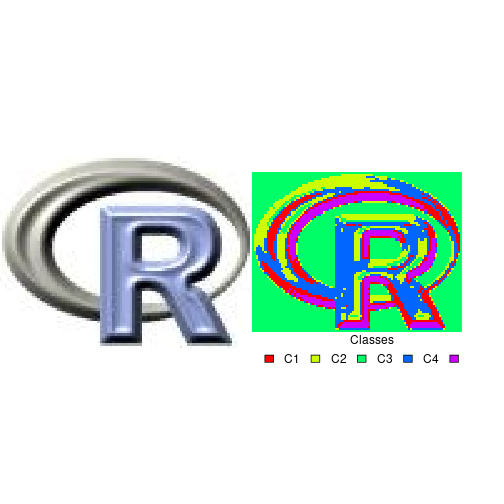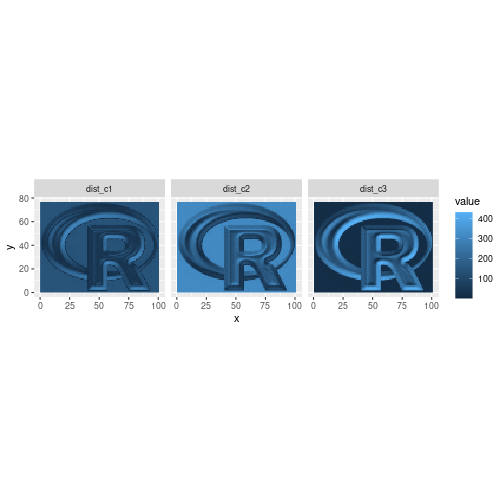unsuperClass { RStoolbox} R Documentation

### Unsupervised Classification

R: Unsupervised Classification

### Description

Unsupervised clustering of Raster* data using kmeans clustering

### Usage

```unsuperClass(
img,
nSamples = 10000,
nClasses = 5,
nStarts = 25,
nIter = 100,
norm = FALSE,
clusterMap = TRUE,
algorithm = "Hartigan-Wong",
output = "classes",
...
)
```

### Arguments

 `img` Raster* object. `nSamples` Integer. Number of random samples to draw to fit cluster map. Only relevant if clusterMap = TRUE. `nClasses` Integer. Number of classes. `nStarts` Integer. Number of random starts for kmeans algorithm. `nIter` Integer. Maximal number of iterations allowed. `norm` Logical. If `TRUE` will normalize img first using normImage. Normalizing is beneficial if your predictors have different scales. `clusterMap` Logical. Fit kmeans model to a random subset of the img (see Details). `algorithm` Character. kmeans algorithm. One of c("Hartigan-Wong", "Lloyd", "MacQueen") `output` Character. Either 'classes' (kmeans class; default) or 'distances' (euclidean distance to each cluster center). `...` further arguments to be passed to writeRaster, e.g. filename

### Details

Clustering is done using `kmeans`. This can be done for all pixels of the image (`clusterMap=FALSE`), however this can be slow and is not memory safe. Therefore if you have large raster data (> memory), as is typically the case with remote sensing imagery it is advisable to choose clusterMap=TRUE (the default). This means that a kmeans cluster model is calculated based on a random subset of pixels (`nSamples`). Then the distance of *all* pixels to the cluster centers is calculated in a stepwise fashion using `predict`. Class assignment is based on minimum euclidean distance to the cluster centers.

The solution of the kmeans algorithm often depends on the initial configuration of class centers which is chosen randomly. Therefore, kmeans is usually run with multiple random starting configurations in order to find a convergent solution from different starting configurations. The `nStarts` argument allows to specify how many random starts are conducted.

### Value

Returns an RStoolbox::unsuperClass object, which is a list containing the kmeans model (\$model) and the raster map (\$map). For output = "classes", \$map contains a RasterLayer with discrete classes (kmeans clusters); for output = "distances" \$map contains a RasterBrick, with 'nClasses' layers, where each layer maps the euclidean distance to the corresponding class centroid.

### Examples

``````library(raster)
input <- brick(system.file("external/rlogo.grd", package="raster"))

## Plot
olpar <- par(no.readonly = TRUE) # back-up par
par(mfrow=c(1,2))
plotRGB(input)

## Run classification
set.seed(25)
unC <- unsuperClass(input, nSamples = 100, nClasses = 5, nStarts = 5)
unC
``````
``````#> unsuperClass results
#>
#> *************** Model ******************
#> \$model
#> K-means clustering with 5 clusters of sizes 14, 21, 40, 16, 9
#>
#> Cluster centroids:
#>         red     green      blue
#> 1  99.57143 100.42857  95.85714
#> 2 189.66667 193.57143 200.28571
#> 3 252.00000 253.17500 252.20000
#> 4 139.31250 145.75000 166.12500
#> 5  26.11111  29.88889  35.44444
#>
#> Within cluster sum of squares by cluster:
#>  11978.571 20760.095  2340.175 17366.188  8932.000
#>
#> *************** Map ******************
#> \$map
#> class      : RasterLayer
#> dimensions : 77, 101, 7777  (nrow, ncol, ncell)
#> resolution : 1, 1  (x, y)
#> extent     : 0, 101, 0, 77  (xmin, xmax, ymin, ymax)
#> crs        : +proj=merc +lon_0=0 +k=1 +x_0=0 +y_0=0 +datum=WGS84 +units=m +no_defs
#> source     : memory
#> names      : class
#> values     : 1, 5  (min, max)
``````
``````## Plots
colors <- rainbow(5)
plot(unC\$map, col = colors, legend = FALSE, axes = FALSE, box = FALSE)
legend(1,1, legend = paste0("C",1:5), fill = colors,
title = "Classes", horiz = TRUE,  bty = "n")
````````````## Return the distance of each pixel to each class centroid
unC <- unsuperClass(input, nSamples = 100, nClasses = 3, output = "distances")
unC
``````
``````#> unsuperClass results
#>
#> *************** Model ******************
#> \$model
#> K-means clustering with 3 clusters of sizes 32, 27, 41
#>
#> Cluster centroids:
#>         red     green      blue
#> 1 164.34375 169.40625 182.34375
#> 2  69.48148  72.40741  77.37037
#> 3 248.41463 248.95122 248.48780
#>
#> Within cluster sum of squares by cluster:
#>   63980.16 115075.56  20710.10
#>
#> *************** Map ******************
#> \$map
#> class      : RasterBrick
#> dimensions : 77, 101, 7777, 3  (nrow, ncol, ncell, nlayers)
#> resolution : 1, 1  (x, y)
#> extent     : 0, 101, 0, 77  (xmin, xmax, ymin, ymax)
#> crs        : +proj=merc +lon_0=0 +k=1 +x_0=0 +y_0=0 +datum=WGS84 +units=m +no_defs
#> source     : memory
#> names      :   dist_c1,   dist_c2,   dist_c3
#> min values : 3.6005371, 1.7153387, 0.7793437
#> max values :  298.2559,  315.1340,  430.6190
``````
``````ggR(unC\$map, 1:3, geom_raster = TRUE)
````````````par(olpar) # reset par
``````

[Package RStoolbox version 0.3.0 Index]